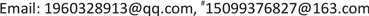﻿ 共同效应结构下的分位数信度模型 Quantile Credibility Models under Common Effect Structure

Statistics and Application
Vol. 08  No. 02 ( 2019 ), Article ID: 29637 , 7 pages
10.12677/SA.2019.82030

Quantile Credibility Models under Common Effect Structure

Ji Cheng*, Lijun Wu#

College of Mathematics and System Sciences, Xinjiang University, Urumqi XinjiangReceived: Mar. 19th, 2019; accepted: Apr. 3rd, 2019; published: Apr. 10th, 2019ABSTRACT

In non-life insurance application research, the classical Bühlmann credibility model cannot effectively reflect the tail information of distribution, and the policy between the risks is not completely independent. Therefore, considering the common effect between risks and combining the credibility theory from the perspective of quantile, the quantile credibility models under common effect structure are studied, which further expands the classical credibility model.

Keywords:Common Effect, Orthogonal Projection, Quantile, Credibility ModelCopyright © 2019 by authors and Hans Publishers Inc.

This work is licensed under the Creative Commons Attribution International License (CC BY).

http://creativecommons.org/licenses/by/4.0/1. 引言

Bühlmann (1967)  提出无分布信度模型，用经验数据的线性组合 ${\alpha }_{0}+\sum _{i=1}^{n}{\alpha }_{i}{X}_{i}$ 来逼近 ${\mu }_{n+1}\left(\theta \right)$ ，通过求解下面的期望损失函数：

2. 模型假设与准备

2.1. 准备知识$\stackrel{^}{\xi }\left(p\right)=n\left(\frac{j}{n}-p\right){X}_{\left(j-1\right)}+n\left(p-\frac{j-1}{n}\right){X}_{\left(j\right)},其中\frac{j-1}{n}\le p\le \frac{j}{n},j=1,\cdots ,n.$

$X={\left({X}_{1}{}^{\prime },{X}_{2}{}^{\prime },\cdots ,{X}_{k}{}^{\prime }\right)}^{\prime }$ 为所有索赔数据组成的列向量， ${\stackrel{^}{\xi }}_{ij}\left(p\right)$ 为样本分位数的估计。我们的目标是通过对样本选取不同分位点，预测每份保单在各分位点第 $n+1$ 年的索赔。由于各保单之间存在某一特定效应，我们用随机变量 $\Lambda$ 来刻画此种共同效应，下面给出模型的基本假设。

2.2. 模型假设

$E\left({\stackrel{^}{\xi }}_{ij}\left(p\right)|{\Theta }_{i},\Lambda \right)={\Xi }_{p}\left({\Theta }_{i},\Lambda \right),Var\left({\stackrel{^}{\xi }}_{ij}\left(p\right)|{\Theta }_{i},\Lambda \right)={V}_{p}\left({\Theta }_{i},\Lambda \right).$

$\begin{array}{l}E\left[{\Xi }_{P}\left({\Theta }_{i},\Lambda \right)|\Lambda \right]={\Xi }_{1}\left(p,\Lambda \right),Var\left[{\Xi }_{P}\left({\Theta }_{i},\Lambda \right)|\Lambda \right]={S}_{2}^{2}\left(p,\Lambda \right),\\ E\left[{\Xi }_{1}\left(p,\Lambda \right)\right]={\Xi }_{1}\left(p\right),E\left[{S}_{2}^{2}\left(p,\Lambda \right)\right]={S}_{2}^{2}\left(p\right),Var\left[{\Xi }_{1}\left(p,\Lambda \right)\right]={\psi }_{1}\left(p\right),\\ E\left[{V}_{p}\left({\Theta }_{i},\Lambda \right)|\Lambda \right]={S}_{1}^{2}\left(p,\Lambda \right),E\left[{S}_{1}^{2}\left(p,\Lambda \right)\right]={S}_{1}^{2}\left(p\right).\end{array}$

2.3. 模型求解

$\underset{A,B}{\mathrm{min}}E\left[\left(\Xi \left(p\right)-A-B\stackrel{^}{\xi }\left(p\right)\right){\left(\Xi \left(p\right)-A-B\stackrel{^}{\xi }\left(p\right)\right)}^{\prime }\right]$ (1)

$\begin{array}{l}\Xi \left(p\right)={\left[{\stackrel{^}{\xi }}_{1,n+1}\left(p\right),\cdots ,{\stackrel{^}{\xi }}_{K,n+1}\left(p\right)\right]}^{\prime }\\ \stackrel{^}{\xi }\left(p\right)={\left[{\stackrel{^}{\xi }}_{1}{\left(p\right)}^{\prime },\cdots ,{\stackrel{^}{\xi }}_{K}{\left(p\right)}^{\prime }\right]}^{\prime }\\ {\stackrel{^}{\xi }}_{i}\left(p\right)={\left[{\stackrel{^}{\xi }}_{i1}\left(p\right),\cdots ,{\stackrel{^}{\xi }}_{in}\left(p\right)\right]}^{\prime }\end{array}$

$\text{pro}\left(Y|L\left(X,1\right)\right)=E\left(Y\right)+Cov\left(Y,X\right)Co{v}^{-1}\left(X,X\right)\left(X-E\left(X\right)\right)$

${\left(A+BCD\right)}^{-1}={A}^{-1}-{A}^{-1}B{\left({C}^{-1}+D{A}^{-1}B\right)}^{-1}D{A}^{-1}$

$\begin{array}{l}\left(A+BCD\right)\left[{A}^{-1}-{A}^{-1}B{\left({C}^{-1}+D{A}^{-1}B\right)}^{-1}D{A}^{-1}\right]\\ \text{ }\text{\hspace{0.17em}}=I-B{\left({C}^{-1}+D{A}^{-1}B\right)}^{-1}D{A}^{-1}+BCD{A}^{-1}-BCD{A}^{-1}B{\left({C}^{-1}+D{A}^{-1}B\right)}^{-1}D{A}^{-1}\\ \text{ }\text{\hspace{0.17em}}=I+BCD{A}^{-1}-B\left(I+CD{A}^{-1}B\right){\left({C}^{-1}+D{A}^{-1}B\right)}^{-1}{C}^{-1}CD{A}^{-1}\\ \text{ }\text{\hspace{0.17em}}=I+BCD{A}^{-1}-B\left(I+CD{A}^{-1}B\right){\left(I+CD{A}^{-1}B\right)}^{-1}CD{A}^{-1}\\ \text{ }\text{\hspace{0.17em}}=I+BCD{A}^{-1}-BCD{A}^{-1}\\ \text{ }\text{\hspace{0.17em}}=I\end{array}$

${\left(A+BCD\right)}^{-1}={A}^{-1}-{A}^{-1}B{\left({C}^{-1}+D{A}^{-1}B\right)}^{-1}D{A}^{-1}$

1) ${\stackrel{^}{\xi }}_{i}\left(p\right)={\left[{\stackrel{^}{\xi }}_{i1}\left(p\right),\cdots ,{\stackrel{^}{\xi }}_{in}\left(p\right)\right]}^{\prime }$ 的期望为：

$E\left[{\stackrel{^}{\xi }}_{i}\left(p\right)\right]={\Xi }_{1}\left(p\right){1}_{n}.$

2) 向量 $\stackrel{^}{\xi }\left(p\right)={\left[{\stackrel{^}{\xi }}_{1}{\left(p\right)}^{\prime },\cdots ,{\stackrel{^}{\xi }}_{K}{\left(p\right)}^{\prime }\right]}^{\prime }$ 的协方差矩阵为：

$Cov\left[\stackrel{^}{\xi }\left(p\right),\stackrel{^}{\xi }\left(p\right)\right]={I}_{k}\otimes \left({S}_{1}^{2}\left(p\right){I}_{n}+{S}_{2}^{2}\left(p\right){1}_{n}{{1}^{\prime }}_{n}\right)+{\psi }_{1}\left(p\right){1}_{nk}{{1}^{\prime }}_{nk}.$

3) 矩阵 $Cov\left[\stackrel{^}{\xi }\left(p\right),\stackrel{^}{\xi }\left(p\right)\right]$ 的逆矩阵为：

$\begin{array}{c}Co{v}^{-1}\left[\stackrel{^}{\xi }\left(p\right),\stackrel{^}{\xi }\left(p\right)\right]=\frac{1}{{S}_{1}^{2}\left(p\right)}{I}_{k}\otimes \left({I}_{n}-\frac{{S}_{2}^{2}\left(p\right)}{{S}_{1}^{2}\left(p\right)+n{S}_{2}^{2}\left(p\right)}{1}_{n}{{1}^{\prime }}_{n}\right)\\ -\frac{{\psi }_{1}\left(p\right)}{\left[{S}_{1}^{2}\left(p\right)+n{S}_{2}^{2}\left(p\right)\right]\left[{S}_{1}^{2}\left(p\right)+n{S}_{2}^{2}\left(p\right)+nk{\psi }_{1}\left(p\right)\right]}{1}_{nk}{{1}^{\prime }}_{nk}.\end{array}$

4) $\Xi \left(p\right)={\left[{\stackrel{^}{\xi }}_{1,n+1}\left(p\right),\cdots ,{\stackrel{^}{\xi }}_{K,n+1}\left(p\right)\right]}^{\prime }$$\stackrel{^}{\xi }\left(p\right)={\left[{\stackrel{^}{\xi }}_{1}{\left(p\right)}^{\prime },\cdots ,{\stackrel{^}{\xi }}_{K}{\left(p\right)}^{\prime }\right]}^{\prime }$ 的协方差矩阵为

$Cov\left[\Xi \left(p\right),\stackrel{^}{\xi }\left(p\right)\right]=\left({S}_{2}^{2}\left(p\right){{e}^{\prime }}_{i}+{\psi }_{1}\left(p\right){{1}^{\prime }}_{k}\right)\otimes {{1}^{\prime }}_{n}.$

1)

$E\left[{\stackrel{^}{\xi }}_{i}\left(p\right)\right]=E\left[\left[\left(E{\stackrel{^}{\xi }}_{i}\left(p\right){|\Theta }_{i},\Lambda \right)\right]=E\left(\begin{array}{c}E\left({\Xi }_{p}\left({\Theta }_{i},\Lambda \right)|\Lambda \right)\\ ⋮\\ E\left({\Xi }_{p}\left({\Theta }_{i},\Lambda \right)|\Lambda \right)\end{array}\right)=E\left(\begin{array}{c}{\Xi }_{1}\left(p,\Lambda \right)\\ ⋮\\ {\Xi }_{1}\left(p,\Lambda \right)\end{array}\right)={\Xi }_{1}\left(p\right){1}_{n}.$

2) 记 $\Theta ={\left({\Theta }_{1},\cdots ,{\Theta }_{k}\right)}^{\prime }$ ，则

$\begin{array}{l}E\left[\stackrel{^}{\xi }\left(p\right)|\Theta ,\Lambda \right]\text{=[}{\Xi }_{p}\left({\Theta }_{1},\Lambda \right){\text{1 ′}}_{n}\text{,}\cdots \text{,}{\Xi }_{p}\left({\Theta }_{k},\Lambda \right){\text{1 ′}}_{n}\text{] ′}={\left[{\Xi }_{p}\left({\Theta }_{1},\Lambda \right)\text{,}\cdots \text{,}{\Xi }_{p}\left({\Theta }_{k},\Lambda \right)\right]}^{\prime }\otimes {\text{1}}_{n}\\ Cov\left[\stackrel{^}{\xi }\left(p\right)|\Theta ,\Lambda \right]=\text{diag}\left[{V}_{p}\left({\Theta }_{1},\Lambda \right){I}_{n}\text{,}\cdots \text{,}{V}_{p}\left({\Theta }_{k},\Lambda \right){I}_{n}\right]\\ Cov\left[\stackrel{^}{\xi }\left(p\right),\stackrel{^}{\xi }\left(p\right)\right]=Cov\left[E\left(\stackrel{^}{\xi }\left(p\right)|\Theta ,\Lambda \right)\right]+E\left[Cov\left(\stackrel{^}{\xi }\left(p\right)|\Theta ,\Lambda \right)\right]\triangleq {Ι}_{1}+{Ι}_{2}\end{array}$

$\begin{array}{c}{Ι}_{1}=Cov\left[E\left(\stackrel{^}{\xi }\left(p\right)|\Theta ,\Lambda \right)\right]\\ =E\left(Cov\left\{{\left[{\Xi }_{p}\left({\Theta }_{1},\Lambda \right),\cdots ,{\Xi }_{p}\left({\Theta }_{k},\Lambda \right)\right]}^{\prime }\otimes {1}_{n}|\Lambda \right\}\right)+Cov\left(E\left\{{\left[{\Xi }_{p}\left({\Theta }_{1},\Lambda \right),\cdots ,{\Xi }_{p}\left({\Theta }_{k},\Lambda \right)\right]}^{\prime }\otimes {1}_{n}|\Lambda \right\}\right)\\ =E\left[{S}_{2}^{2}\left(p,\Lambda \right)\right]{I}_{k}\otimes {1}_{n}{{1}^{\prime }}_{n}+Cov\left[{\Xi }_{1}\left(p,\Lambda \right){1}_{nk}\right]\\ ={S}_{2}^{2}\left(p\right){I}_{k}\otimes {1}_{n}{{1}^{\prime }}_{n}+{\psi }_{1}\left(p\right){1}_{nk}{{1}^{\prime }}_{nk}\\ {Ι}_{2}=E\left[Cov\left(\stackrel{^}{\xi }\left(p\right)|\Theta ,\Lambda \right)\right]={S}_{1}^{2}\left(p\right){I}_{nk}\end{array}$

$Cov\left[\stackrel{^}{\xi }\left(p\right),\stackrel{^}{\xi }\left(p\right)\right]\triangleq {Ι}_{1}+{Ι}_{2}={I}_{k}\otimes \left({S}_{1}^{2}\left(p\right){I}_{n}+{S}_{2}^{2}\left(p\right){1}_{n}{{1}^{\prime }}_{n}\right)+{\psi }_{1}\left(p\right){1}_{nk}{{1}^{\prime }}_{nk}$

3) 根据引理2得

$\begin{array}{c}{\left({S}_{1}^{2}\left(p\right){I}_{n}\text{+}{S}_{2}^{2}\left(p\right){1}_{n}{{1}^{\prime }}_{n}\right)}^{-1}=\frac{1}{{S}_{1}^{2}\left(p\right)}{I}_{n}-\frac{\left(p\right){1}_{n}{{1}^{\prime }}_{n}}{\frac{1}{{S}_{2}^{2}\left(p\right)}+\frac{1}{{S}_{1}^{2}\left(p\right)}{1}_{n}{{1}^{\prime }}_{n}}\\ =\frac{1}{{S}_{1}^{2}\left(p\right)}\left({I}_{n}-\frac{{S}_{2}^{2}\left(p\right)}{{S}_{1}^{2}\left(p\right)+n{S}_{2}^{2}\left(p\right)}{1}_{n}{{1}^{\prime }}_{n}\right)\end{array}$

$\begin{array}{c}Co{v}^{-1}\left[\stackrel{^}{\xi }\left(p\right),\stackrel{^}{\xi }\left(p\right)\right]={I}_{k}\otimes {\left({S}_{1}^{2}\left(p\right){I}_{n}\text{+}{S}_{2}^{2}\left(p\right){1}_{n}{{1}^{\prime }}_{n}\right)}^{-1}\\ -\frac{\left[{I}_{k}\otimes {\left({S}_{1}^{2}\left(p\right){I}_{n}\text{+}{S}_{2}^{2}\left(p\right){1}_{n}{{1}^{\prime }}_{n}\right)}^{-1}\right]{1}_{nk}{{1}^{\prime }}_{nk}\left[{I}_{k}\otimes {\left({S}_{1}^{2}\left(p\right){I}_{n}\text{+}{S}_{2}^{2}\left(p\right){1}_{n}{{1}^{\prime }}_{n}\right)}^{-1}\right]}{\frac{1}{{\psi }_{1}\left(p\right)}+{{1}^{\prime }}_{nk}\left[{I}_{k}\otimes {\left({S}_{1}^{2}\left(p\right){I}_{n}\text{+}{S}_{2}^{2}\left(p\right){1}_{n}{{1}^{\prime }}_{n}\right)}^{-1}\right]{1}_{nk}}\\ =\frac{1}{{S}_{1}^{2}\left(p\right)}{I}_{k}\otimes \left({I}_{n}-\frac{{S}_{2}^{2}\left(p\right)}{{S}_{1}^{2}\left(p\right)+n{S}_{2}^{2}\left(p\right)}{1}_{n}{{1}^{\prime }}_{n}\right)\\ -\frac{{\psi }_{1}\left(p\right)}{\left[{S}_{1}^{2}\left(p\right)+n{S}_{2}^{2}\left(p\right)\right]\left[{S}_{1}^{2}\left(p\right)+n{S}_{2}^{2}\left(p\right)+nk{\psi }_{1}\left(p\right)\right]}{1}_{nk}{{1}^{\prime }}_{nk}.\end{array}$

4) 因为 $Cov\left(\Xi \left(p\right),\stackrel{^}{\xi }\left(p\right)|\Theta ,\Lambda \right)=0$ ，则

$\begin{array}{c}Cov\left[\Xi \left(p\right),\stackrel{^}{\xi }\left(p\right)\right]=Cov\left[E\left(\Xi \left(p\right)|\Theta ,\Lambda \right),E\left(\stackrel{^}{\xi }\left(p\right)|\Theta ,\Lambda \right)\right]+E\left[Cov\left(\Xi \left(p\right),\stackrel{^}{\xi }\left(p\right)|\Theta ,\Lambda \right)\right]\\ =Cov\left\{{\Xi }_{p}\left({\Theta }_{i},\Lambda \right),{\left[{\Xi }_{p}\left({\Theta }_{1},\Lambda \right),\cdots ,{\Xi }_{p}\left({\Theta }_{k},\Lambda \right)\right]}^{\prime }\otimes {1}_{n}\right\}\\ =E\left(Cov\left\{{\Xi }_{p}\left({\Theta }_{i},\Lambda \right),{\left[{\Xi }_{p}\left({\Theta }_{1},\Lambda \right),\cdots ,{\Xi }_{p}\left({\Theta }_{k},\Lambda \right)\right]}^{\prime }\otimes {1}_{n}|\Lambda \right\}\right)\\ +Cov\left(E\left[{\Xi }_{p}\left({\Theta }_{i},\Lambda \right)|\Lambda \right],{\left\{E\left[{\Xi }_{p}\left({\Theta }_{1},\Lambda \right)|\Lambda \right],\cdots ,E\left[{\Xi }_{p}\left({\Theta }_{k},\Lambda \right)|\Lambda \right]\right\}}^{\prime }\otimes {1}_{n}\right)\\ ={S}_{2}^{2}\left(p\right){{e}^{\prime }}_{i}\otimes {{1}^{\prime }}_{n}+Cov\left[{\Xi }_{1}\left(p,\Lambda \right),{\Xi }_{1}\left(p,\Lambda \right){1}_{nk}\right]={S}_{2}^{2}\left(p\right){{e}^{\prime }}_{i}\otimes {{1}^{\prime }}_{n}+{\psi }_{1}\left(p\right){{1}^{\prime }}_{nk}\\ =\left({S}_{2}^{2}\left(p\right){{e}^{\prime }}_{i}+{\psi }_{1}\left(p\right){{1}^{\prime }}_{k}\right)\otimes {{1}^{\prime }}_{n}\end{array}$

3. 具有共同效应结构的分位数信度模型

$\stackrel{^}{\Xi }\left(p\right)={Z}_{1}\overline{\stackrel{^}{\xi }}\left(p\right)+{Z}_{2}\overline{\overline{\stackrel{^}{\xi }}}\left(p\right)+{Z}_{3}{\Xi }_{1}\left(p\right)$

$\begin{array}{l}{Z}_{1}=\frac{n{S}_{2}^{2}\left(p\right)}{n{S}_{2}^{2}\left(p\right)+{S}_{1}^{2}\left(p\right)},\\ {Z}_{2}=\frac{nk{\psi }_{1}\left(p\right){S}_{1}^{2}\left(p\right)}{\left[n{S}_{2}^{2}\left(p\right)+{S}_{1}^{2}\left(p\right)\right]\left[nk{\psi }_{1}\left(p\right)+n{S}_{2}^{2}\left(p\right)+{S}_{1}^{2}\left(p\right)\right]},\\ {Z}_{3}=1-{Z}_{1}-{Z}_{2}=\frac{{S}_{1}^{2}\left(p\right)}{nk{\psi }_{1}\left(p\right)+n{S}_{2}^{2}\left(p\right)+{S}_{1}^{2}\left(p\right)}.\end{array}$

${\overline{\stackrel{^}{\xi }}}_{i}\left(p\right)=\frac{1}{n}\sum _{j=1}^{n}{\stackrel{^}{\xi }}_{ij}\left(p\right),\overline{\overline{\stackrel{^}{\xi }}}\left(p\right)=\frac{1}{k}\sum _{i=1}^{k}{\overline{\stackrel{^}{\xi }}}_{i}\left(p\right).$

$\begin{array}{c}\stackrel{^}{\Xi }\left(p\right)=E\left[\Xi \left(p\right)\right]+Cov\left[\Xi \left(p\right),\stackrel{^}{\xi }\left(p\right)\right]Co{v}^{-1}\left[\stackrel{^}{\xi }\left(p\right),\stackrel{^}{\xi }\left(p\right)\right]\left[\stackrel{^}{\xi }\left(p\right)-E\left(\stackrel{^}{\xi }\left(p\right)\right)\right]\\ ={\Xi }_{1}\left(p\right)+\frac{1}{n{S}_{2}^{2}\left(p\right)+{S}_{1}^{2}\left(p\right)}\left[{S}_{2}^{2}\left(p\right){{e}^{\prime }}_{i}\otimes {{1}^{\prime }}_{n}+\frac{{\psi }_{1}\left(p\right){S}_{1}^{2}\left(p\right)}{nk{\psi }_{1}\left(p\right)+n{S}_{2}^{2}\left(p\right)+{S}_{1}^{2}\left(p\right)}{{1}^{\prime }}_{nk}\right]\left[\stackrel{^}{\xi }\left(p\right)-{\Xi }_{1}\left(p,\Lambda \right){1}_{nk}\right]\\ ={\Xi }_{1}\left(p\right)+\frac{1}{n{S}_{2}^{2}\left(p\right)+{S}_{1}^{2}\left(p\right)}\left[n{S}_{2}^{2}\left(p\right)\left(\overline{{\stackrel{^}{\xi }}_{i}}\left(p\right)-{\Xi }_{1}\left(p\right)\right)+\frac{nk{\psi }_{1}\left(p\right){S}_{1}^{2}\left(p\right)}{nk{\psi }_{1}\left(p\right)+n{S}_{2}^{2}\left(p\right)+{S}_{1}^{2}\left(p\right)}\left(\overline{\overline{\stackrel{\wedge }{\xi }}}\left(p\right)-{\Xi }_{1}\left(p\right)\right)\right]\\ ={Z}_{1}\overline{{\stackrel{^}{\xi }}_{i}}\left(p\right)+{Z}_{2}\overline{\overline{\stackrel{^}{\xi }}}\left(p\right)+{Z}_{3}{\Xi }_{1}\left(p\right)\end{array}$

4. 总结

Quantile Credibility Models under Common Effect Structure[J]. 统计学与应用, 2019, 08(02): 270-276. https://doi.org/10.12677/SA.2019.82030

1. 1. Bühlmann, H. (1967) Experience Rating and Credibility. ASTIN Bulletin, 5, 199-207. https://doi.org/10.1017/S0515036100008989

2. 2. Bühlmann, H. and Straub, E. (1970) Glaubwürdigkeit für schadensätze. Bulletin of the Swiss Association of Actuaries, 70, 111-133.

3. 3. Yeo, K.L. and Valdez, E.A. (2006) Claim Dependence with Common Effects in Credibility Models. Insurance Mathematics & Economics, 38, 609-629. https://doi.org/10.1016/j.insmatheco.2005.12.006

4. 4. Wen, L., Wu, X. and Zhou, X. (2009) The Credibility Premiums for Models with Dependence Induced by Common Effects. Insurance Mathematics & Economics, 44, 19-25. https://doi.org/10.1016/j.insmatheco.2008.09.005

5. 5. Wang, Z. and Wen, L. (2011) Regression Credibility Models with Random Common Effects. Chinese Journal of Applied Probability & Statistics, 27, 312-322.

6. 6. Zhang, Q. and Chen, P. (2018) Credibility Estimators with Dependence Structure over Risks and Time under Balanced Loss Function. Statistica Neerlandica, 72, 153-179.

7. 7. Pitselis, G. (2013) Quantile Credibility Models. Insurance Mathematics & Economics, 52, 477-489. https://doi.org/10.1016/j.insmatheco.2013.02.011

8. NOTES

*第一作者。

#通讯作者。